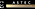Algebra I

Algebra I emphasizes the study of multiple representations of linear and non-linear functions. It includes mathematical concepts for working with rational numbers, various expressions, analyzing and solving linear equations and inequalities, data analysis, probability, statistics and polynomials. Scholars use hands-on materials and calculators when needed in solving problems where the algebra concepts are applied.

Geometry

Prerequisite:  The successful completion of Algebra I

Geometry requires scholars to reason, justify, prove, define, apply and explain theorems about points, lines, circles, polygons, right triangles and three-dimensional shapes.  Scholars connect algebra and geometry through the coordinate plane and solve multi-step contextual problems through applying geometric concepts in modeling situations.

Algebra II

Prerequisite:  The successful completion of Algebra I and Geometry

Algebra II guides scholars in reasoning, solving, interpreting, graphing, creating and analyzing linear, quadratic, logarithmic, rational, radical and exponential functions. Scholars expand concepts including complex numbers, probability, linear inequalities, systems of equations and systems of inequalities. Scholars make inferences and draw conclusions from data using samples, experiments and hypothesis testing, which prepares scholars for more advanced mathematics.

.

Trigonometry/Precalculus

Prerequisite:  The successful completion of Algebra I, Geometry and Algebra II

Trigonometry/Precalculus is an advanced math course where scholars study trigonometric functions, identities, trigonometric equations and their variations.  Scholars conduct an in-depth analysis of polynomials (solving and graphing), series and sequences (finite and infinite), math induction, probability, logic, vectors and complex numbers.  Trigonometry/Precalculus prepares scholars for success in college mathematics.